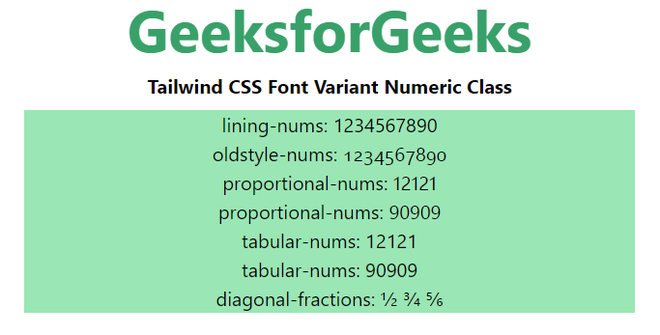GFG App
Open AppBrowser
Continue

# Tailwind CSS Font Variant Numeric

This class accepts lots of values in tailwind CSS in which all the properties are covered in class form.  It is the alternative to the CSS font-variant-numeric property. This class is used to control the usage of alternate glyphs. This is done in terms of units or markers such as numbers or fractions.

Font variant numeric classes:

• normal-nums: Using normal-nums will remove each effect of the font-variant-numeric class.
• ordinal: This value directly indicates the open type values i.e. ordn. The term makes use of special glyphs for ordinal markers.
• slashed-zero: This class slashed-zero used a zero with a slash, which proves to be very useful while distinguishing between 0 and O.
• lining-nums: This class corresponds to the open type values i.e lnum. This keyword activates the numbers lying on the baseline.
• oldstyle-nums: This class corresponds to the open type values i.e onum. This keyword activates the set of figures where some numbers have descendant.
• proportional-nums: This class activates those norms where not every number is of the same size. Its open type value is pnum.
• tabular-nums: This class open type value is tnum. It activates those sets of figures where a set of numbers is of the same size.
• diagonal-fractions: It’s open type value is frac. This activates those sets of figures where numerator and denominator are made smaller and are separated by a slash.
• stacked-fractions: It’s open type value is arac. This activates those set of figures where numerator and denominator are made smaller, stacked, and are separated by a horizontal line.

Syntax:

`<element class="Font-Variant-Numeric">...</element>`

Example:

## HTML

 `  ` `<``head``>  ` `    ``<``link` `href``= ` `"https://unpkg.com/tailwindcss@^1.0/dist/tailwind.min.css"`  `          ``rel``=``"stylesheet"``>  ` `  ` ` `  `<``body` `class``=``"text-center mx-4 space-y-2"``>  ` `    ``<``h1` `class``=``"text-green-600 text-5xl font-bold"``> ` `        ``GeeksforGeeks ` `    ``  ` `    ``<``b``>Tailwind CSS Font Variant Numeric Class  ` `    ``<``div` `class``=``"bg-green-300 mx-24 h-full"``> ` `        ``<``p` `class``=``"lining-nums"``>lining-nums: 1234567890 ` ` `  ` `  `        ``<``p` `class``=``"oldstyle-nums"``>oldstyle-nums: 1234567890 ` ` `  ` `  `        ``<``p` `class``=``"proportional-nums"``>proportional-nums: 12121 ` ` `  ` `  `        ``<``p` `class``=``"proportional-nums"``>proportional-nums: 90909 ` ` `  ` `  `        ``<``p` `class``=``"tabular-nums"``>tabular-nums: 12121 ` ` `  ` `  `        ``<``p` `class``=``"tabular-nums"``>tabular-nums: 90909 ` ` `  ` `  `        ``<``p` `class``=``"diagonal-fractions"``> ` `            ``diagonal-fractions: 1/2 3/4 5/6 ` `        `` ` ` `  `    `` ` `  ` ` `  ``

Output:My Personal Notes arrow_drop_up